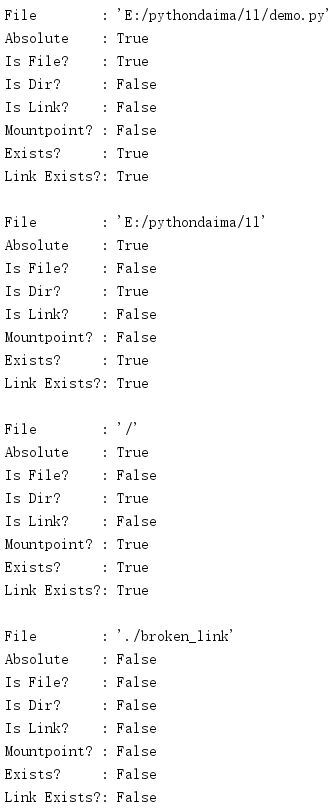# 1. os.path平台独立的文件名管理

## 1.1 解析路径

os.path中的第一组函数可以用来将表示文件名的字符串解析为文件名的各个组成部分。这些函数并不要求路径真正存在：它们只是处理字符串。

os.sep：路径各部分之间的分隔符(例如，“/”或“\”)。

os.extsep：文件名与文件“扩展名”之间的分隔符(例如“.”)。

os.pardir：路径中表示目录树上一级的部分(例如：“..”)。

os.curdir：路径中指示当前目录的部分(例如：“.”)。

split()函数将路径分解为两个单独的部分，并返回包含这些结果的一个tuple。这个tuple的第二个元素是路径的最后一部分，第一个元素则是此前的所有内容。

import os.path

PATHS = [
'/one/two/three',
'/one/two/three/',
'/',
'.',
'',
]

for path in PATHS:
print('{!r:>17} : {}'.format(path, os.path.split(path)))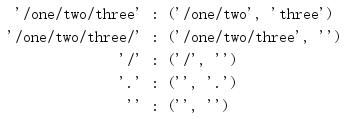basename()函数返回的值等价于split()值的第二部分。

import os.path

PATHS = [
'/one/two/three',
'/one/two/three/',
'/',
'.',
'',
]

for path in PATHS:
print('{!r:>17} : {!r}'.format(path, os.path.basename(path))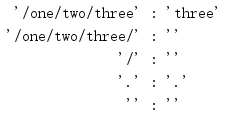dirname()函数返回分解路径得到的第一部分。

import os.path

PATHS = [
'/one/two/three',
'/one/two/three/',
'/',
'.',
'',
]

for path in PATHS:
print('{!r:>17} : {!r}'.format(path, os.path.dirname(path)))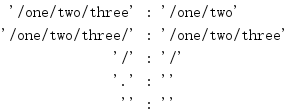splitext()的工作类似于split()，不过它会根据扩展名分隔符而不是目录分隔符来分解路径。

import os.path

PATHS = [
'filename.txt',
'filename',
'/path/to/filename.txt',
'/',
'',
'my-archive.tar.gz',
'no-extension.',
]

for path in PATHS:
print('{!r:>21} : {!r}'.format(path, os.path.splitext(path)))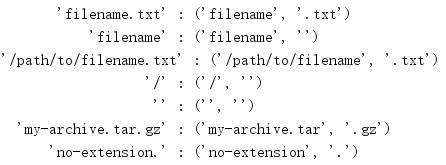commonprefix()取一个路径列表作为参数，并且返回一个字符串，表示所有路径中都出现的公共前缀。这个值可能表示一个根本不存在的路径，而且并不考虑路径分隔符，所以这个前缀可能并不落在一个分隔符边界上。

import os.path

paths = ['/one/two/three/four',
'/one/two/threefold',
'/one/two/three/',
]
for path in paths:
print('PATH:', path)

print()
print('PREFIX:', os.path.commonprefix(paths))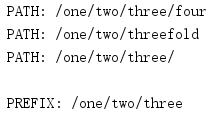commonpath()则要考虑路径分隔符。它返回的前缀不包括部分路径值。

import os.path

paths = ['/one/two/three/four',
'/one/two/threefold',
'/one/two/three/',
]
for path in paths:
print('PATH:', path)

print()
print('PREFIX:', os.path.commonpath(paths))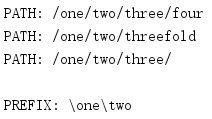## 1.2 建立路径

import os.path

PATHS = [
('one', 'two', 'three'),
('/', 'one', 'two', 'three'),
('/one', '/two', '/three'),
]

for parts in PATHS:
print('{} : {!r}'.format(parts, os.path.join(*parts)))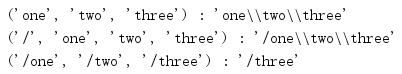import os.path

for user in ['', 'dhellmann', 'nosuchuser']:
lookup = '~' + user
print('{!r:>15} : {!r}'.format(
lookup, os.path.expanduser(lookup)))expandvars()更为通用，它会扩展路径中出现的所有shell环境变量。

import os.path
import os

os.environ['MYVAR'] = 'VALUE'

print(os.path.expandvars('/path/to/\$MYVAR'))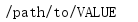## 1.3 规范化路径

import os.path

PATHS = [
'one//two//three',
'one/./two/./three',
'one/../alt/two/three',
]

for path in PATHS:
print('{!r:>22} : {!r}'.format(path, os.path.normpath(path)))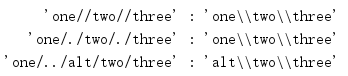import os
import os.path

os.chdir('/')

PATHS = [
'.',
'..',
'./one/two/three',
'../one/two/three',
]

for path in PATHS:
print('{!r:>21} : {!r}'.format(path, os.path.abspath(path)))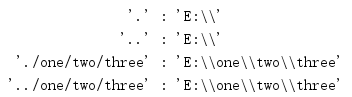## 1.4 文件时间

import os.path
import time

print('File         :', __file__)
print('Access time  :', time.ctime(os.path.getatime(__file__)))
print('Modified time:', time.ctime(os.path.getmtime(__file__)))
print('Change time  :', time.ctime(os.path.getctime(__file__)))
print('Size         :', os.path.getsize(__file__))

os.path.getatime()返回访问时间，os.path.getmtime()返回修改时间，os.path.getctime()返回创建时间。os.path.getsize()返回文件中的数据量，以字节为单位表示。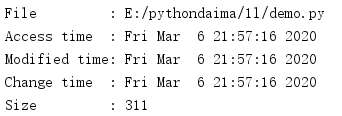## 1.5 测试文件

import os.path

FILENAMES = [
__file__,
os.path.dirname(__file__),
'/',
]

for file in FILENAMES:
print('File        : {!r}'.format(file))
print('Absolute    :', os.path.isabs(file))
print('Is File?    :', os.path.isfile(file))
print('Is Dir?     :', os.path.isdir(file))
print()